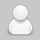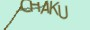# Python内置函数

watrt4个月前 (07-27)Python1410

1. `abs()`: 返回一个数的绝对值。

• 参数：abs(x)

2. `all()`: 如果可迭代对象中的所有元素都为 True，则返回 True。

• 参数：all(iterable)

3. `any()`: 如果可迭代对象中的任何元素为 True，则返回 True。

• 参数：any(iterable)

4. `ascii()`: 返回一个包含 ASCII 转义字符的字符串表示。

• 参数：ascii(object)

5. `bin()`: 将一个整数转换为二进制字符串。

• 参数：bin(x)

6. `bool()`: 将给定参数转换为布尔值。

• 参数：bool(x)

7. `bytearray()`: 返回一个新的字节数组对象。

• 参数：bytearray(source, encoding, errors)

8. `bytes()`: 返回一个新的字节对象。

• 参数：bytes(source, encoding, errors)

9. `callable()`: 检查对象是否可调用（函数、方法等）。

• 参数：callable(object)

10. `chr()`: 返回一个 Unicode 字符对应的字符串。

• 参数：chr(i)

11. `classmethod()`: 用于将方法转换为类方法。

• 参数：classmethod(function)

12. `compile()`: 将源代码编译为代码对象或 AST 对象。

• 参数：compile(source, filename, mode, flags=0, dont_inherit=False, optimize=-1)

13. `complex()`: 创建一个复数对象。

• 参数：complex(real=0, imag=0)

14. `delattr()`: 从对象中删除指定的属性。

• 参数：delattr(object, name)

15. `dict()`: 创建字典或将其他映射类型对象转换为字典。

• 参数：dict(**kwargs), dict(mapping, **kwargs), dict(iterable, **kwargs)

16. `dir()`: 返回对象的属性列表。

• 参数：dir([object])

17. `divmod()`: 返回两个数的商和余数。

• 参数：divmod(a, b)

18. `enumerate()`: 返回一个枚举对象，包含可迭代对象中的索引和值。

• 参数：enumerate(iterable, start=0)

19. `eval()`: 执行一个字符串表达式，并返回表达式的值。

• 参数：eval(expression, globals=None, locals=None)

20. `exec()`: 执行一个字符串或代码对象，并返回 None。

• 参数：exec(object, globals=None, locals=None)

21. `filter()`: 返回一个迭代器，包含满足指定条件的元素。

• 参数：filter(function, iterable)

22. `float()`: 将对象转换为浮点数。

• 参数：float(x=0)

23. `format()`: 对值进行格式化，并返回格式化后的字符串。

• 参数：format(value, format_spec)

24. `frozenset()`: 创建一个不可变的集合。

• 参数：frozenset(iterable)

25. `getattr()`: 返回对象指定的属性值。

• 参数：getattr(object, name[, default])

26. `globals()`: 返回当前全局符号表的字典。

• 参数：globals()

27. `hasattr()`: 检查对象是否具有指定的属性。

• 参数：hasattr(object, name)

28. `hash()`: 返回对象的哈希值。

• 参数：hash(object)

29. `help()`: 显示对象的帮助文档。

• 参数：help([object])

30. `hex()`: 将一个整数转换为十六进制字符串。

• 参数：hex(x)

31. `id()`: 返回对象的唯一标识符。

• 参数：id(object)

32. `input()`: 从控制台获取用户输入，并返回输入值。

• 参数：input(prompt)

33. `int()`: 将对象转换为整数。

• 参数：int(x=0)

34. `isinstance()`: 检查一个对象是否属于指定的类型。

• 参数：isinstance(object, classinfo)

35. `issubclass()`: 检查一个类是否是另一个类的子类。

• 参数：issubclass(class, classinfo)

36. `iter()`: 返回一个迭代器对象。

• 参数：iter(iterable, sentinel)

37. `len()`: 返回对象的长度或元素个数。

• 参数：len(s)

38. `list()`: 将可迭代对象转换为列表。

• 参数：list(iterable)

39. `locals()`: 返回当前局部符号表的字典。

• 参数：locals()

40. `map()`: 将函数应用于可迭代对象的每个元素，并返回一个迭代器。

• 参数：map(function, iterable, ...)

41. `max()`: 返回给定参数的最大值。

• 参数：max(iterable, *[, key, default])

42. `memoryview()`: 返回一个内存视图对象。

• 参数：memoryview(obj)

43. `min()`: 返回给定参数的最小值。

• 参数：min(iterable, *[, key, default])

44. `next()`: 返回可迭代对象的下一个元素。

• 参数：next(iterator, default)

45. `object()`: 创建一个基类对象。

• 参数：object()

46. `oct()`: 将一个整数转换为八进制字符串。

• 参数：oct(x)

47. `open()`: 打开文件，并返回文件对象。

• 参数：open(file, mode='r', buffering=-1, encoding=None, errors=None, newline=None, closefd=True, opener=None)

48. `ord()`: 返回一个字符的整数表示。

• 参数：ord(c)

49. `pow()`: 返回 x 的 y 次幂的结果。

• 参数：pow(x, y[, z])

50. `print()`: 输出内容到控制台。

• 参数：print(value, ..., sep=' ', end='\n', file=sys.stdout, flush=False)

51. `property()`: 返回属性的属性对象。

• 参数：property([get[, set[, delete[, doc]]]])

52. `range()`: 返回一个序列的整数。

• 参数：range(stop), range(start, stop[, step])

53. `repr()`: 返回一个对象的字符串表示。

• 参数：repr(object)

54. `reversed()`: 返回一个反向迭代器。

• 参数：reversed(seq)

55. `round()`: 对一个数字进行四舍五入。

• 参数：round(number[, ndigits])

56. `set()`: 创建一个集合或将可迭代对象转换为集合。

• 参数：set(iterable)

57. `setattr()`: 设置对象的属性值。

• 参数：setattr(object, name, value)

58. `slice()`: 返回一个切片对象。

• 参数：slice(stop), slice(start, stop[, step])

59. `sorted()`: 对可迭代对象进行排序。

• 参数：sorted(iterable, key=None, reverse=False)

60. `staticmethod()`: 将方法转换为静态方法。

• 参数：staticmethod(function)

61. `str()`: 将对象转换为字符串。

• 参数：str(object)

62. `sum()`: 返回可迭代对象中所有元素的总和。

• 参数：sum(iterable, start=0)

63. `super()`: 返回一个超类对象。

### micropython中使用rtc设置时间

micropython中使用rtc设置时间from machine import RTC rtc = RTC() rtc.datetime((2017, 8, 23, 1, 12, 48, 0, 0)) # set a specific date and time print(rtc.datetime(...

### micropython 使用oled显示前面设置的时间。

from machine import I2C, Pin import ssd1306,time    #这里引用的是官方的ssd1306库。可以在github上下载下来放入库中。 i2c = I2C(-1, Pin(14), Pin(2)) display = ssd1306.SSD1306_I2C(128, 64, i...

### micropython连接到wifi上面

import network,time,ujson,urequests,ntptime from machine import RTC nic=network.WLAN(network.STA_IF) nic.active(True) #nic.connect('ZCWH','00004157') nic.connect('028sd.com','88884444') nic.co...

#### 发表评论◎欢迎参与讨论，请在这里发表您的看法和观点。